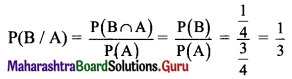Balbharti Maharashtra State Board Class 11 Maths Solutions Pdf Chapter 9 Probability Miscellaneous Exercise 9 Questions and Answers.

## Maharashtra State Board 11th Maths Solutions Chapter 9 Probability Miscellaneous Exercise 9

(I) Select the correct answer from the given four alternatives.

Question 1.
There are 5 girls and 2 boys, then the probability that no two boys are sitting together for a photograph is
(A) $$\frac{1}{21}$$
(B) $$\frac{4}{7}$$
(C) $$\frac{2}{7}$$
(D) $$\frac{5}{7}$$
(D) $$\frac{5}{7}$$
Hint:
There are 5 girls and 2 boys.
They can be arranged among themselves in $${ }^{7} \mathrm{P}_{7}$$ = 7! ways.
∴ Girls can be arranged among themselves in $${ }^{5} \mathrm{P}_{5}$$ = 5! ways.
No two boys should sit together.
Let girls be denoted by the letter G.
– G – G – G – G – G –
There are 6 places, marked by ‘-’ where boys can sit.
∴ Boys can be arranged in
$${ }^{6} \mathrm{P}_{2}=\frac{6 !}{(6-2) !}$$
= $$\frac{6 \times 5 \times 4 !}{4 !}$$
= 30 ways.
∴ Required probability = $$\frac{5 ! \times 30}{7 !}=\frac{5 ! \times 30}{7 \times 6 \times 5 !}=\frac{5}{7}$$

Question 2.
In a jar, there are 5 black marbles and 3 green marbles. Two marbles are picked randomly one after the other without replacement. What is the possibility that both the marbles are black?
(A) $$\frac{5}{14}$$
(B) $$\frac{5}{8}$$
(C) $$\frac{5}{7}$$
(D) $$\frac{5}{16}$$
(A) $$\frac{5}{14}$$Question 3.
Two dice are thrown simultaneously. Then the probability of getting two numbers whose product is even is
(A) $$\frac{3}{4}$$
(B) $$\frac{1}{4}$$
(C) $$\frac{5}{7}$$
(D) $$\frac{1}{2}$$
(A) $$\frac{3}{4}$$
Hint:
Two dice are thrown.
∴ n(S) = 36
Getting two numbers whose product is even, i.e., one of the two numbers must be even.
Let event A: Getting even number on first dice,
event B: Getting even number on second dice.
n(A) = 18, n(B) = 18, n(A ∩ B) = 9
Required probability = P(A ∩ B)
= $$\frac{n(A)+n(B)-n(A \cap B)}{n(S)}$$
= $$\frac{18+18-9}{36}$$
= $$\frac{3}{4}$$

Question 4.
In a set of 30 shirts, 17 are white and the rest are black. 4 white and 5 black shirts are tagged as ‘PARTY WEAR’. If a shirt is chosen at random from this set, the possibility of choosing a black shirt or a ‘PARTY WEAR’ shirt is
(A) $$\frac{11}{15}$$
(B) $$\frac{13}{30}$$
(C) $$\frac{9}{13}$$
(D) $$\frac{17}{30}$$
(D) $$\frac{17}{30}$$
Hint:
17 white + 13 black = 30 shirts
4 white and 5 black are ‘PARTY WEAR’
A: Choosing a black shirt
∴ P(A) = $$\frac{{ }^{13} C_{1}}{{ }^{30} C_{1}}=\frac{13}{30}$$
B: Choosing a ‘PARTY WEAR’ shirt.
∴ P(B) = $$\frac{{ }^{9} \mathrm{C}_{1}}{{ }^{30} \mathrm{C}_{1}}=\frac{9}{30}$$
There are 5 black ‘PARTY WEAR’ shirts.
∴ P(A ∩ B) = $$\frac{{ }^{5} \mathrm{C}_{1}}{{ }^{30} \mathrm{C}_{1}}=\frac{5}{30}$$
∴ Required probability
P(A ∪ B) = P(A) + P(B) – P(A ∩ B)
= $$\frac{13}{30}$$ + $$\frac{9}{30}$$ – $$\frac{5}{30}$$
= $$\frac{17}{30}$$

Question 5.
There are 2 shelves. One shelf has 5 Physics and 3 Biology books and the other has 4 Physics and 2 Biology books. The probability of drawing a Physics book is
(A) $$\frac{9}{14}$$
(B) $$\frac{31}{48}$$
(C) $$\frac{9}{38}$$
(D) $$\frac{1}{2}$$
(B) $$\frac{31}{48}$$
Hint:
Let event S1: First shelve is selected,
event S2: Second shelve is selected,
event P: Drawing a physics book.
∴ P(S1) = $$\frac{1}{2}$$ and P(S2) = $$\frac{1}{2}$$
First shelve has 5 physics and 3 biology books, i.e., total 8 books.
∴ P(P/S1) = $$\frac{{ }^{5} C_{1}}{{ }^{8} C_{1}}=\frac{5}{8}$$
Similarly, P(P/S2) = $$\frac{{ }^{4} C_{1}}{{ }^{6} C_{1}}=\frac{4}{6}=\frac{2}{3}$$
∴ P(P) = P(S1) . P(P/S1) + P(S2) . P(P/S2)
= $$\frac{1}{2} \times \frac{5}{8}+\frac{1}{2} \times \frac{2}{3}$$
= $$\frac{31}{48}$$Question 6.
Two friends A and B apply for a job in the same company. The chances of A getting selected is 2/5 and that of B is 4/7. The probability that both of them get selected is
(A) $$\frac{34}{35}$$
(B) $$\frac{1}{35}$$
(C) $$\frac{8}{35}$$
(D) $$\frac{27}{35}$$
(C) $$\frac{8}{35}$$

Question 7.
The probability that a student knows the correct answer to a multiple-choice question is $$\frac{2}{3}$$. If the student does not know the answer, then the student guesses the answer. The probability of the guessed answer being correct is $$\frac{1}{4}$$. Given that the student has answered the question correctly, the probability that the student knows the correct answer is
(A) $$\frac{5}{6}$$
(B) $$\frac{6}{7}$$
(C) $$\frac{7}{8}$$
(D) $$\frac{8}{9}$$
(D) $$\frac{8}{9}$$
Hint:
Let event A: Student knows the correct answer,
event A’: Student guesses the answer,
∴ P(A) = $$\frac{2}{3}$$, P(A’) = $$\frac{1}{3}$$, P(B/A’) = $$\frac{1}{4}$$
Clearly, P(B/A) = 1
Required probability = P(A/B)
= $$\frac{\mathrm{P}(\mathrm{A}) \cdot \mathrm{P}(\mathrm{B} / \mathrm{A})}{\mathrm{P}(\mathrm{A}) \cdot \mathrm{P}(\mathrm{B} / \mathrm{A})+\mathrm{P}\left(\mathrm{A}^{\prime}\right) \mathrm{P}\left(\mathrm{B} / \mathrm{A}^{\prime}\right)}$$
= $$\frac{\frac{2}{3} \times 1}{\frac{2}{3} \times 1+\frac{1}{3} \times \frac{1}{4}}$$
= $$\frac{8}{9}$$

Question 8.
The bag I contain 3 red and 4 black balls while Bag II contains 5 red and 6 black balls. One ball is drawn at random from one of the bags and it is found to be red. The probability that it was drawn from Bag II is
(A) $$\frac{33}{68}$$
(B) $$\frac{35}{69}$$
(C) $$\frac{34}{67}$$
(D) $$\frac{35}{68}$$
(D) $$\frac{35}{68}$$

Question 9.
A fair die is tossed twice. What are the odds in favour of getting 4, 5, or 6 on the first toss and 1, 2, 3, or 4 on the second toss?
(A) 1 : 3
(B) 3 : 1
(C) 1 : 2
(D) 2 : 1
(C) 1 : 2
Hint:
A fair dice is tossed twice.
∴ n(S) = 36
A: Getting 4, 5, or 6 on the first toss and Getting 1, 2, 3, or 4 on the second toss.
∴ A = {(4, 1), (4, 2), (4, 3), (4, 4), (5, 1), (5, 2), (5, 3), (5, 4), (6, 1), (6, 2), (6, 3), (6, 4)}
∴ P(A) = $$\frac{n(A)}{n(S)}=\frac{12}{36}=\frac{1}{3}$$
∴ Required answer = P(A) : P(A’) = 1 : 2Question 10.
The odds against an event are 5 : 3 and the odds in favour of another independent event are 7 : 5. The probability that at least one of the two events will occur is
(A) $$\frac{52}{96}$$
(B) $$\frac{71}{96}$$
(C) $$\frac{69}{96}$$
(D) $$\frac{13}{96}$$
(B) $$\frac{71}{96}$$

(II) Solve the following.

Question 1.
The letters of the word ‘EQUATION’ are arranged in a row. Find the probability that
(i) all the vowels are together
(ii) arrangement starts with a vowel and ends with a consonant.
Solution:
The letters of the word EQUATION can be arranged in 8! ways.
∴ n(S) = 8!
There are 5 vowels and 3 consonants.
(i) A: all vowels are together we need to arrange (E, U, A, I, O), Q, T, N
Let us consider all vowels as one unit.
So, there are 4 units, which can be arranged in 4! ways.
Also, 5 vowels can be arranged among themselves in 5! ways.
∴ n(A) = 4! × 5!
Required probability = P(A)
= $$\frac{n(A)}{n(S)}$$
= $$\frac{4 ! \times 5 !}{8 !}$$
= $$\frac{1}{14}$$

(ii) B: arrangement start with a vowel and ends with a consonant.
First and last places can be filled in 5 and 3 ways respectively.
Remaining 6 letters are arranged in 6! Ways.
∴ n(B) = 5 × 3 × 6!
Required probability = P(B)
= $$\frac{n(B)}{n(S)}$$
= $$\frac{5 \times 3 \times 6 !}{8 !}$$
= $$\frac{15}{56}$$Question 2.
There are 6 positive and 8 negative numbers. Four numbers are chosen at random, without replacement, and multiplied. Find the probability that the product is a positive number.
Solution:
Let event A: Four positive numbers are chosen,
event B: Four negative numbers are chosen,
event C: Two positive and two negative numbers are chosen.
Since four numbers are chosen without replacement,
n(A) = 6 × 5 × 4 × 3 = 360
n(B) = 8 × 7 × 6 × 5 = 1680
In event C, four numbers are to be chosen without replacement such that two numbers are positive and two numbers ate negative. This can be done in following ways:
+ + – – OR + – + – OR + – – + OR – + – + OR – – + + OR – + + –
∴ n(C) = 6 × 5 × 8 × 7 + 6 × 8 × 5 × 7 + 6 × 8 × 7 × 5 + 8 × 6 × 7 × 5 + 6 × 5 × 8 × 7 + 8 × 6 × 5 × 7
= 6 × (8 × 7 × 6 × 5)
=10080
Here, total number of numbers = 14
∴ n(S) = 14 × 13 × 12 × 11 = 24024
Since A, B, C are mutually exclusive events,
Required probability = P(A) + P(B) + P(C)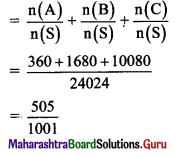Question 3.
Ten cards numbered 1 to 10 are placed in a box, mixed up thoroughly, and then one card is drawn randomly. If it is known that the number on the drawn card is more than 3, what is the probability that it is an even number?
Solution:
S = {1, 2,…., 10}
∴ n(S) = 10
A: Number is more than 3.
A = {4, 5, 6, 7, 8, 9, 10}
∴ n(A) = 7
∴ P(A) = $$\frac{n(A)}{n(S)}=\frac{7}{10}$$
B: Number is even.
B = {2, 4, 6, 8, 10}
∴ A ∩ B = {4, 6, 8, 10}
∴ n(A ∩ B) = 4
∴ P(A ∩ B) = $$\frac{\mathrm{n}(\mathrm{A} \cap \mathrm{B})}{\mathrm{n}(\mathrm{S})}=\frac{4}{10}$$
Required probability = P(B/A)
= $$\frac{P(A \cap B)}{P(A)}$$
= $$\frac{\left(\frac{4}{10}\right)}{\left(\frac{7}{10}\right)}$$
= $$\frac{4}{7}$$Question 4.
If A, B and C are independent events, P(A ∩ B) = $$\frac{1}{2}$$, P(B ∩ C) = $$\frac{1}{3}$$, P(C ∩ A) = $$\frac{1}{6}$$, then find P(A), P(B) and P(C).
Solution:
Since A and B are independent events,
P(A ∩ B) = P(A) . P(B)
∴ P(A) . P(B) = $$\frac{1}{2}$$ ……(i)
B and C are independent events.
∴ P(B ∩ C) = P(B) . P(C)
∴ P(B) . P(C) = $$\frac{1}{3}$$ ……(ii)
A and C are independent events.
∴ P(A ∩ C) = P(A) . P(C)
∴ P(A) . P(C) = $$\frac{1}{6}$$ ……(iii)
Dividing (i) by (ii), we get
$$\frac{\mathrm{P}(\mathrm{A}) \mathrm{P}(\mathrm{B})}{\mathrm{P}(\mathrm{B}) \mathrm{P}(\mathrm{C})}=\frac{\frac{1}{2}}{\frac{1}{3}}$$
P(A) = $$\frac{3}{2}$$ P(C) ……(iv)
Substituting equation (iv) in (iii), we get
$$\frac{3}{2}$$ P(C) . P(C) = $$\frac{1}{6}$$
[P(C)]2 = $$\frac{1}{9}$$
∴ P(C) = $$\frac{1}{3}$$
Substituting P(C) = $$\frac{1}{3}$$ in equation (ii), we get P(B) = 1
Substituting P(B) = 1 in equation (i), we get P(A) = $$\frac{1}{2}$$

Question 5.
If the letters of the word ‘REGULATIONS’ be arranged at random, what is the probability that there will be exactly 4 letters between R and E?
Solution:
There are 11 letters in the word ‘REGULATIONS’ which can be arranged among themselves in 11! ways.
∴ n(S) = 11!
Let event A: There will be exactly 4 letters between R and E.
R, E can occur at (1, 6), (2, 7), ….,(6, 11) positions. So, there are 6 possibilities.
Also, R and E can interchange their positions.
So, R, E can be arranged in 2 × 6 = 12 ways.
Remaining 9 letters can be arranged in 9! ways.
∴ n(A) = 12 × 9!
∴ P(A) = $$\frac{\mathrm{n}(\mathrm{A})}{\mathrm{n}(\mathrm{S})}=\frac{12 \times 9 !}{11 !}=\frac{12 \times 9 !}{11 \times 10 \times 9 !}=\frac{6}{55}$$

Question 6.
In how many ways can the letters of the word ARRANGEMENTS be arranged?
(i) Find the chance that an arrangement chosen at random begins with the letters EE.
(ii) Find the probability that the consonants are together.
Solution:
The word ‘ARRANGEMENTS’ has 12 letters in which 2A, 2E, 2N, 2R, G, M, T, S are there.
n(S) = Total number of arrangements = $$\frac{12 !}{2 ! 2 ! 2 ! 2 !}=\frac{12 !}{(2 !)^{4}}$$
(i) A: Arrangement chosen at random begins with the letters EE.
If the first and second places are filled with EE, there are 10 letters left in which 2A, 2N, 2R, G, M, T, S are there.
∴ n(A) = $$\frac{10 !}{2 ! 2 ! 2 !}=\frac{10 !}{(2 !)^{3}}$$

(ii) B: Consonants (G, M, T, S, 2N, 2R) are together.
2A, 2E, and the group containing consonants form total 5 units. Which can be arranged in $$\frac{5 !}{2 ! 2 !}$$ ways.
Also, 8 consonants can be arranged among themselves in $$\frac{8 !}{2 ! 2 !}$$ ways.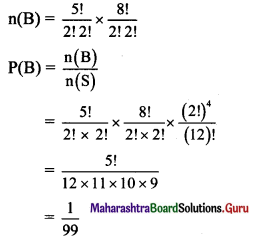Question 7.
A letter is taken at random from the letters of the word ‘ASSISTANT’ and another letter is taken at random from the letters of the word ‘STATISTICS’. Find the probability that the selected letters are the same.
Solution:
Word ASSISTANT has 2A, I, N, 3S, 2T, and word STATISTICS has A, C, 2I, 3S, 3T.
C and N are uncommon letters.
In the words ASSISTANT, there are 9 letters out of which 2 letters are ‘A’, and in the word STATISTICS, there are 10 letters, out of which 1 letter is A.
∴ Probability of choosing A from both the letters = $$\frac{{ }^{2} C_{1}}{{ }^{9} C_{1}} \times \frac{{ }^{1} C_{1}}{{ }^{10} C_{1}}=\frac{2}{9} \times \frac{1}{10}=\frac{1}{45}$$
Similarly,
Probability of choosing I from both the letters = $$\frac{{ }^{1} \mathrm{C}_{1}}{{ }^{9} \mathrm{C}_{1}} \times \frac{{ }^{2} \mathrm{C}_{1}}{{ }^{10} \mathrm{C}_{1}}=\frac{1}{9} \times \frac{2}{10}=\frac{1}{45}$$
Probability of choosing S from both the letters = $$\frac{{ }^{3} \mathrm{C}_{1}}{{ }^{9} \mathrm{C}_{1}} \times \frac{{ }^{3} \mathrm{C}_{1}}{{ }^{10} \mathrm{C}_{1}}=\frac{3}{9} \times \frac{3}{10}=\frac{1}{10}$$
Probability of choosing T from both the letters = $$\frac{{ }^{2} C_{1}}{{ }^{9} C_{1}} \times \frac{{ }^{3} C_{1}}{{ }^{10} C_{1}}=\frac{2}{9} \times \frac{3}{10}=\frac{1}{15}$$
Required probability = $$\frac{1}{45}+\frac{1}{45}+\frac{1}{10}+\frac{1}{15}$$ = $$\frac{19}{90}$$

Question 8.
A die is loaded in such a way that the probability of the face with j dots turning up is proportional to j for j = 1, 2,….., 6. What is the probability, in one roil of the die, that an odd number of dots will turn up?
Solution:
According to the given condition, the probability of the face with 1, 2, 3, 4, 5, 6 dots turning up is proportional to 1, 2, 3, 4, 5, 6.
Let k be the common ration of proportionality.
∴ The probabilities of the faces with 1, 2, 3, 4, 5, 6 dots turning up are 1k , 2k, 3k, 4k, 5k, 6k respectively.
Since sum of the probabilities = 1,
k(1 + 2+ ….. + 6) = 1
k($$\frac{6 \times 7}{2}$$) = 1
k = $$\frac{1}{21}$$
Required probability = P(1) + P(3) + P(5)
= $$\frac{1}{21}+\frac{3}{21}+\frac{5}{21}$$
= $$\frac{9}{21}$$
= $$\frac{3}{7}$$

Question 9.
An urn contains 5 red balls and 2 green balls. A ball is drawn. If it’s green, a red ball is added to the urn, and if it’s red, a green ball is added to the urn. (The original ball is not returned to the urn). Then a second ball is drawn. What is the probability that the second ball is red?
Solution:
A: Event of drawing a red ball and placing a green ball in the urn
B: Event of drawing a green ball and placing a red ball
C: Event of drawing a red ball in the second draw
P(A) = $$\frac{5}{7}$$
P(B) = $$\frac{2}{7}$$
P(C/A) = $$\frac{4}{7}$$
P(C/B) = $$\frac{6}{7}$$
Required probability
P(C) = P(A) P(C/A) + P(B) P(C/B)
= $$\frac{5}{7} \times \frac{4}{7}+\frac{2}{7} \times \frac{6}{7}$$
= $$\frac{32}{49}$$Question 10.
The odds against A solving a certain problem are 4 to 3 and the odds in favour of B solving the same problem are 7 to 5, find the probability that the problem will be solved.
Solution:
The odds against A solving the problems are 4 : 3.
Let P(A’) = P(A does not solve the problem) = $$\frac{4}{4+3}=\frac{4}{7}$$
So, the probability that A solves the problem = P(A) = 1 – P(A’)
= 1 – $$\frac{4}{7}$$
= $$\frac{3}{7}$$
Similarly, let P(B) = P(B solves the problem)
Since odds in favour of B solving the problem are 7 : 5.
∴ P(B) = $$\frac{7}{7+5}=\frac{7}{12}$$
Required probability
P(A ∪ B) = P(A) + P(B) – P(A ∩ B)
Since A and B are independent events.
∴ P(A ∩ B) = P(A) . P(B)
∴ Required probability = P(A) + P(B) – P(A) . P(B)
= $$\frac{3}{7}+\frac{7}{12}-\frac{3}{7} \times \frac{7}{12}$$
= $$\frac{16}{21}$$

Question 11.
If P(A) = P(A/B) = $$\frac{1}{5}$$, P(B/A) = $$\frac{1}{3}$$, then find
(i) P(A’/B)
(ii) P(B’/A’)
Solution:
Since P(A) = P(A/B) = $$\frac{1}{5}$$
P(A) = $$\frac{1}{5}$$
and $$\frac{P(A \cap B)}{P(B)}=\frac{1}{5}$$
∴ P(A) = $$\frac{1}{5}$$ ……(i)
P(B) = 5 P(A ∩ B) ……..(ii)
Since P(B/A) = $$\frac{1}{3}$$
$$\frac{P(A \cap B)}{P(A)}=\frac{1}{3}$$
∴ P(A) = 3 P(A ∩ B) ………(iii)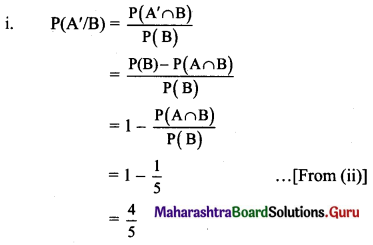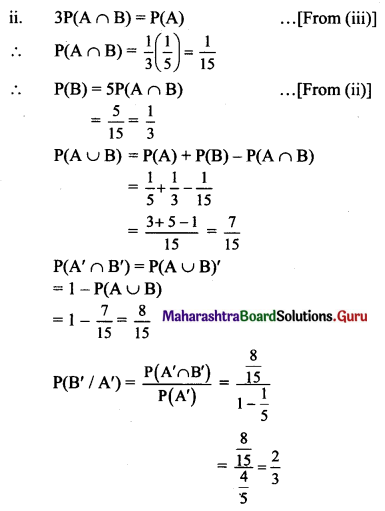Question 12.
Let A and B be independent events with P(A) = $$\frac{1}{4}$$ and P(A ∪ B) = 2P(B) – P(A). Find
(i) P(B)
(ii) P(A/B)
(iii) P(B’/A)
Solution:
A and B are independent events. .
∴ P(A ∩ B) = P(A) × P(B)
(i) P(A ∪ B) = P(A) + P(B) – P(A ∩ B)
∴ P(A ∪ B) = P(A) + P(B) – P(A) × P(B)
∴ 2P(B) – P(A) = P(A) + P(B) – P(A) × P(B) ……[∵ P(A ∪ B) = 2P(B) – P(A)]
∴ 2P(B) – $$\frac{1}{4}$$ = $$\frac{1}{4}$$ + P(B) – $$\frac{1}{4}$$ × P(B)
∴ 2P(B) – P(B) + $$\frac{1}{4}$$ P(B) = $$\frac{1}{4}$$ + $$\frac{1}{4}$$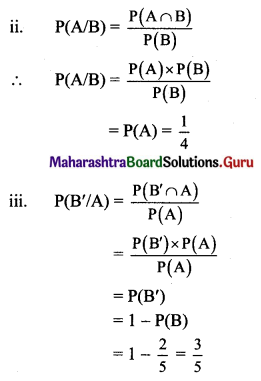Question 13.
Find the probability that a year selected will have 53 Wednesdays.
Solution:
A leap year comes after 3 years.
∴ The probability of a year being a leap year = $$\frac{1}{4}$$
∴ Probability of a year being a non-leap year = 1 – $$\frac{1}{4}$$ = $$\frac{3}{4}$$
In a non-leap year, there are 52 weeks and one extra day, whereas a leap year has 52 weeks and 2 extra days.
∴ 53rd Wednesday’s chance in a non-leap year = $$\frac{1}{7}$$
Two extra days of a leap year can be
(Mon, Tue), (Tue, Wed), (Wed, Thu), (Thu, Fri), (Fri, Sat), (Sat, Sun), (Sun, Mon)
∴ There are 2 possibilities of 53rd Wednesday in a leap year.
∴ 53rd Wednesday’s chance in a leap year = $$\frac{2}{7}$$
Required probability = P(a non-leap year and Wednesday) + P(a leap year and Wednesday)
= $$\frac{3}{4} \times \frac{1}{7}+\frac{1}{4} \times \frac{2}{7}$$
= $$\frac{5}{28}$$

Question 14.
The chances of P, Q and R, getting selected as principal of a college are $$\frac{2}{5}$$, $$\frac{2}{5}$$, $$\frac{1}{5}$$ respectively. Their chances of introducing IT in the college are $$\frac{1}{2}$$, $$\frac{1}{3}$$, $$\frac{1}{4}$$ respectively. Find the probability that
(a) IT is introduced in the college after one of them is selected as a principal.
(b) IT is introduced by Q.
Solution:
Let event P: P become principal,
event Q: Q become principal,
event R: R become principal,
event E: Subject IT is introduced.
Given, P(P) = $$\frac{2}{5}$$
P(Q) = $$\frac{2}{5}$$
P(R) = $$\frac{1}{5}$$
P(E/P) = $$\frac{1}{2}$$
P(E/Q) = $$\frac{1}{3}$$
P(E/R) = $$\frac{1}{4}$$
(a) Required probability
P(E) = P(P) P(E/P) + P(Q) P(E/Q) + P(R) P(E/R)
= $$\frac{2}{5} \times \frac{1}{2}+\frac{2}{5} \times \frac{1}{3}+\frac{1}{5} \times \frac{1}{4}$$
= $$\frac{1}{5}+\frac{2}{15}+\frac{1}{20}$$
= $$\frac{12+8+3}{60}$$
= $$\frac{23}{60}$$

(b) Required probability = P(Q/E)
By Bayes’ theorem,
P(Q/E) = $$\frac{P(Q) P(E / Q)}{P(E)}$$
= $$\frac{\frac{2}{5} \times \frac{1}{3}}{\frac{23}{60}}$$
= $$\frac{8}{23}$$Question 15.
Suppose that five good fuses and two defective ones have been mixed up. To find the defective fuses, we test them one-by-one, at random and without replacement What is the probability that we are lucky and find both of the defective fuses in the first two tests?
Solution:
Number of fuses = 5 + 2 = 7
Testing two fuses one-by-one at random, without replacement from 7 can be done in $${ }^{7} \mathrm{C}_{1} \times{ }^{6} \mathrm{C}_{1}$$ ways.
∴ n(S) = $${ }^{7} \mathrm{C}_{1} \times{ }^{6} \mathrm{C}_{1}$$ = 7 × 6 = 42
Let event A: Getting defective fuses in the first two tests without replacement.
There are two defective fuses.
∴ n(A) = $${ }^{2} \mathrm{C}_{1} \times{ }^{1} \mathrm{C}_{1}$$ = 2 × 1 = 2
∴ P(A) = $$\frac{n(A)}{n(S)}=\frac{2}{42}=\frac{1}{21}$$

Question 16.
For three events A, B and C, we know that A and C are independent, B and C are independent, A and B are disjoint, P(A ∪ C) = $$\frac{2}{3}$$, P(B ∪ C) = $$\frac{3}{4}$$, P(A ∪ B ∪ C) = $$\frac{11}{12}$$. Find P(A), P(B) and P(C).
Solution:
Let P(A) = x, P(B) = y, P(C) = z
Since A, B are disjoint,
A ∩ B = Φ and A ∩ B ∩ C = Φ
∴ P(A ∩ B) = 0, P(A ∩ B ∩ C) = 0 ……(i)
Since A and C are independent,
P(A ∩ C) = P(A) P(C) = xz
Since B and C are independent,
P(B ∩ C) = P(B) P(C) = yz
P(A ∪ C) = P(A) + P(C) – P(A ∩ C)
∴ $$\frac{2}{3}$$ = x + z – xz ……..(ii)
P(B ∪ C) = P(B) + P(C) – P(B ∩ C)
∴ $$\frac{3}{4}$$ = y + z – yz ………(iii)
P(A ∪ B ∪ C) = P(A) + P(B) + P(C) – P(A ∩ B) – P(B ∩ C) – P(C ∩ A) + P(A ∩ B ∩ C)
$$\frac{11}{12}$$ = x + y + z – 0 – yz – zx + 0 …… [From(i)]
= (x + z – xz) + (y + z – yz) – z
= $$\frac{2}{3}+\frac{3}{4}$$ – z ……. [From (ii) and (iii)]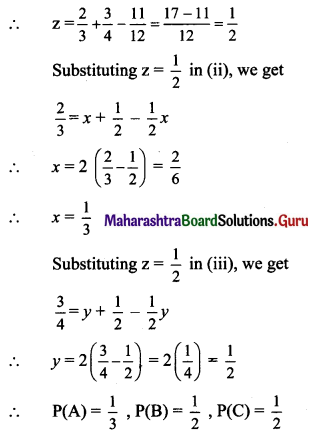Question 17.
The ratio of boys to girls in a college is 3 : 2 and 3 girls out of 500 and 2 boys out of 50 of that college are good singers. A good singer is chosen. What is the probability that the chosen singer is a girl?
Solution:
Let event S: The student is a good singer,
event B: The student is a boy,
event G: The student is a girl.
Since the ratio of boys to girls is 3 : 2 and 3 girls out of 500 and 2 boys out of 50 are good singers.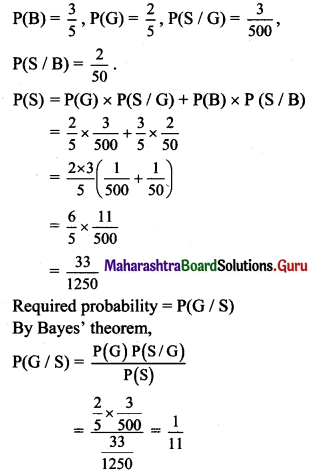Question 18.
A and B throw a die alternatively till one of them gets a 3 and wins the game. Find the respective probabilities of winning. (Assuming A begins the game).
Solution:
Since P(getting 3) = $$\frac{1}{6}$$,
P(not getting 3) = 1 – $$\frac{1}{6}$$ = $$\frac{5}{6}$$
In 1st throw if A gets 3, A wins
∴ P(A win) = $$\frac{1}{6}$$
In 2nd throw by B (i.e., A does not get 3),
∴ P(B wins) = $$\frac{5}{6} \times \frac{1}{6}$$
In 3rd throw by A, P(A wins) = $$\frac{5}{6} \times \frac{5}{6} \times \frac{1}{6}$$
(3rd throw by A shows that B has lost in 2nd throw) and so on.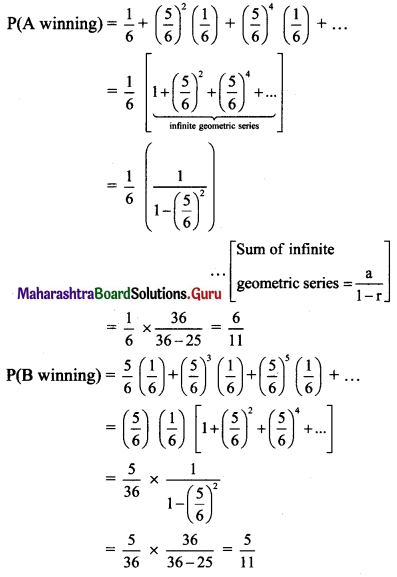Question 19.
Consider independent trials consisting of rolling a pair of fair dice, over and over. What is the probability that a sum of 5 appears before a sum of 7?
Solution:
When two dice are thrown, the sample space is
S = {(1, 1), (1, 2), (1, 3), (1, 4), (1, 5), (1, 6), (2, 1), (2, 2), (2, 3), (2, 4), (2, 5), (2, 6), (3, 1), (3, 2), (3, 3), (3, 4), (3, 5), (3, 6), (4, 1), (4, 2), (4, 3), (4, 4), (4, 5), (4, 6), (5, 1), (5, 2), (5, 3), (5, 4), (5, 5), (5, 6), (6, 1), (6, 2), (6, 3), (6, 4), (6, 5), (6, 6)}
∴ n(S) = 36
Let event A: The sum is 5 in a trial.
A = {(2, 3), (3, 2), (1, 4), (4, 1)}
∴ P(A) = $$\frac{4}{36}=\frac{1}{9}$$
Let event B: The sum is 7 in a trial.
B = {(2, 5), (5, 2), (3, 4), (4, 3), (1, 6), (6, 1)}
∴ P(B) = $$\frac{6}{36}=\frac{1}{6}$$
Let event C: Neither sum is 5 nor 7.
P(C) = 1 – P(A) – P(B)
= 1 – $$\frac{1}{9}$$ – $$\frac{1}{6}$$
= $$\frac{26}{36}$$
Let the sum of 5 appear in the nth trial for the first time and the sum of 7 has not occurred in the first (n – 1) trials.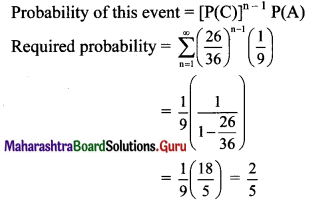Question 20.
A machine produces parts that are either good (90%), slightly defective (2%), or obviously defective (8%). Produced parts get passed through an automatic inspection machine, which is able to detect any part that is obviously defective and discard it. What is the probability that the quality of the parts that make it through the inspection machine and get shipped?
Solution:
Let event G: The event that machine produces a good part,
event S: The event that machine produces a slightly defective part,
event D: The event that machine produces an obviously defective part.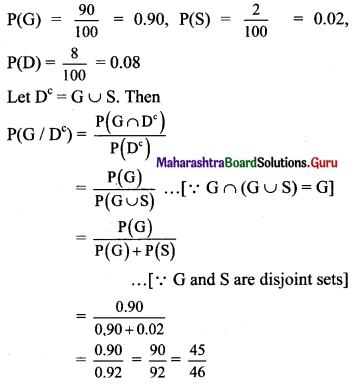Question 21.
Given three identical boxes, I, II, and III, each containing two coins. In box I, both coins are gold coins, in box II, both are silver coins and in box III, there is one gold and one silver coin. A person chooses a box at random and takes out a coin. If the coin is of gold, what is the probability that the other coin in the box is also of gold?
Solution:
Let event B1: Select box I having two gold coins.
event B2: Selecting box II having two silver coins,
event B3: Selecting box III having one silver and one gold coin,
event G: Coin is gold.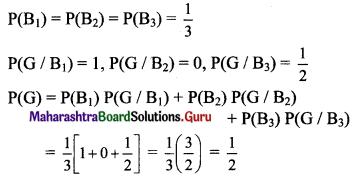To find the probability that the other can in the box is also gold. Which is possible only when it is drawn from the box I.
∴ Required probability = P(B1/G)
By Bayes’ theorem,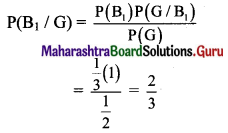Question 22.
In a factory which manufactures bulbs, machines A, B, and C manufacture respectively 25%, 35% and 40% of the bulbs. Of their outputs, 5, 4, and 2 percent are respectively defective bulbs. A bulb is drawn at random from the product and is found to be defective. What is the probability that it is manufactured by machine B?
Solution:
Let event A: Bulb manufactured by machine A
event B: Bulb manufactured by machine B
event C: Bulb manufactured by machine C
event D: Bulb defective
∴ P(A) = $$\frac{25}{100}$$
P(B) = $$\frac{35}{100}$$
P(C) = $$\frac{40}{100}$$
Machines A, B and C manufacture respectively 25%, 35% and 40% of the bulbs.
Of their outputs, 5, 4, and 2 percent are respectively defective bulbs.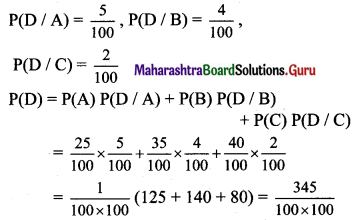Required probability = P(B/D)
By Bayes’ theorem,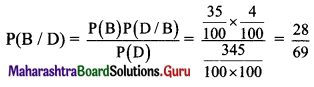Question 23.
A family has two children. One of them is chosen at random and found that the child is a girl. Find the probability that
(i) both the children are girls.
(ii) both the children are girls given that at least one of them is a girl.
Solution:
A family has two children.
∴ Sample space S = {BB, BG, GB, GG}
(i) A: First child is a girl.
∴ A = {GB, GG}
∴ P(A) = $$\frac{2}{4}=\frac{1}{2}$$
B: Second child is a girl.
∴ B = {BG, GG}
∴ A ∩ B = {GG}
∴ P(A ∩ B) = $$\frac{1}{4}$$
Required probability
P(B/A) = $$\frac{\mathrm{P}(\mathrm{A} \cap \mathrm{B})}{\mathrm{P}(\mathrm{A})}=\frac{\frac{1}{4}}{\frac{1}{2}}=\frac{1}{2}$$

(ii) A: At least one of the children is a girl.
∴ A = {GG, GB, BG}
∴ P(A) = $$\frac{3}{4}$$
B: both children are girls.
B = {GG}
∴ P(B) = $$\frac{1}{4}$$
Also, A ∩ B = B